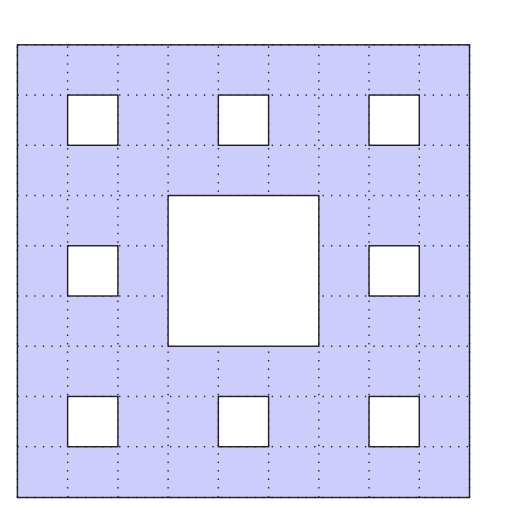# Math help: 2020 AMC Junior Section Q28

• Starting with a 9×9×9 cube, Augusta removed
as few 1 × 1 × 1 cubes as possible so that the
resulting sculpture had front view, top view and
side view all the same, as shown.
How many 1 × 1 × 1 cubes did Augusta remove?• @gentlegorilla This one calls for a bit of 3D visualization! Based on the diagram, you can imagine three sets of 9 "bars" running through the cube. (One set running front to back, one left to right, and one from top to bottom) Can you find the volume of all those "bars"?

Unfortunately, this isn't exactly the answer... if you imagine the cube, some of the bars will "overlap," meaning that we subtracted too much. So the next step would be to figure out what bars overlap, and what the volume of the overlap is.

Hope that helped, and feel free to respond here with any other questions/answers!(By the way, this problem is interesting because, even though it's geometry, it's very similar to a counting and probability technique called PIE. We use PIE to count the number of elements in some sets by first adding up the elements in all the sets, then subtracting and adding to correct for over/undercounting until we get to the right answer.)